Geosci. Model Dev., 15, 1659–1676, 2022
https://doi.org/10.5194/gmd-15-1659-2022
Geosci. Model Dev., 15, 1659–1676, 2022
https://doi.org/10.5194/gmd-15-1659-2022

Model description paper 25 Feb 2022

Model description paper | 25 Feb 2022# The PFLOTRAN Reaction Sandbox

The PFLOTRAN Reaction Sandbox
Glenn E. Hammond Glenn E. Hammond
• Environmental Subsurface Science Group, Pacific Northwest National Laboratory, Richland, WA, USA

Correspondence: Glenn E. Hammond (glenn.hammond@pnnl.gov)

Abstract

As modern reactive transport simulators evolve to accommodate the demands of a user community, researchers need a platform for prototyping new biogeochemical processes, many of which are niche and specific to laboratory or field experiments. The PFLOTRAN Reaction Sandbox leverages modern, object-oriented Fortran in an attempt to provide such an environment within an existing reactive transport simulator. This work describes the PFLOTRAN Reaction Sandbox concept and implementation through several illustrative examples. Reaction Sandbox Biodegradation Hill customizes the existing microbially mediated biodegradation reaction formulation within PFLOTRAN to better match empirical data. Reaction Sandbox Simple provides an isolated environment for testing numerous preconfigured kinetic rate expressions and developing user intuition. Reaction Sandbox Example serves as a template for creating new sandboxes within PFLOTRAN.

Share
1 Introduction

Modern reactive transport simulators incorporate sophisticated networks of reactions to simulate complex biogeochemical processes within the Earth's subsurface environment in support of scientific research in climate change, fate and transport of contaminants, and water resources management . As these simulators mature and evolve over time, they can accumulate a large number of chemical reactions with a wide range of implementations. Many reactions are somewhat common among reactive transport codes (e.g., aqueous complexation, mineral precipitation–dissolution, radioactive decay and sorption; ), while others are niche, prototypical or problem dependent. There is a multitude of possible reactions to include within a simulator, and the approach to implementing these reactions often varies among simulators. For instance, sorption processes include absorption, adsorption and ion exchange. Surface complexation is an adsorptive process that can be simulated using a constant capacitance, (diffuse) double layer, triple layer or non-electrostatic model (Bethke2007). Depending on the reaction timescales, surface complexation may be simulated assuming local equilibrium or driven by a kinetic rate expression, and kinetic approaches may include single and multi-rate models, the latter utilizing a distribution of rate constants associated with size fractions of sediment material .

It is also common for researchers to develop one-off implementations of reactions when existing capabilities cannot replicate biogeochemical phenomena observed in field or laboratory experiments. For example, demonstrated that the brucite silicification is a serpentinization rate-limiting reaction that is exponentially dependent upon aqueous silica activity. Their brucite silicification reaction required the implementation of a custom rate law that was exponentially dependent upon the activity of aqueous silica. These one-off implementations quickly increase the diversity of reactions supported by a simulator. As the number of reactions implemented within a simulator grows, and the reactions become increasingly diverse, adding new reactions can challenge ongoing simulator development and maintenance, especially within an open-source community where code development is often crowd-sourced.

Due to time and funding limitations, it is difficult for code developers to satisfy the needs of the user community by implementing all variants of a reactive process. Nor does it make sense when reactions are problem-specific and may never be used in the future. Meanwhile, the end-users who are requesting customization are often non-computational domain scientists with less interest in or limited understanding of numerical methods, programming paradigms, code abstractions and data layout. Their focus is more on improving predictive accuracy through the refinement of reaction conceptual models, not code development. This raises the question as to what steps can be taken in the design and implementation of a reactive transport simulator to facilitate code development and long-term maintenance while flattening the (often) steep learning curve for new developers.

The PFLOTRAN Reaction Sandbox is an attempt to provide such an environment within an existing reactive transport simulator. The purpose of the Reaction Sandbox is to provide a means for testing alternative implementations for kinetically formulated rate expressions or networks of these reactions in conjunction with the existing reactive transport capability within PFLOTRAN. Within computer science, the term sandbox often refers to an environment for implementing and vetting new, untested algorithms in isolation. The purpose of a sandbox is to limit the impact on the remainder of the code.

The following sections document the implementation of the PFLOTRAN Reaction Sandbox and demonstrate its application on several problem scenarios. Section 2 provides an overview of PFLOTRAN by presenting the governing equations for reactive transport and the numerical methods employed to solve the resulting discrete nonlinear systems of equations. The section also presents PFLOTRAN's conventional reactive transport capability and discusses the code's modular object-oriented design that facilitates the implementation of the Reaction Sandbox. Section 3 describes the foundational (Fortran) Reaction Sandbox class upon which all Reaction Sandbox classes are coded and the high-level programming interface through which the remainder of the code accesses sandbox reactions. Section 4 documents several example sandboxes from which a researcher may derive their own implementation. Finally, Sect. 5 summarizes the approach.

2 Background

PFLOTRAN is an massively parallel, reactive multiphase flow and transport simulator for modeling subsurface earth system processes . The code has been developed under open-source GNU LGPL licensing since 2009 and contains contributions from an international group of developers. PFLOTRAN is written in Fortran 2003/2008, which facilitates object-oriented design through the use of nested derived types, classes (extensible derived types with member procedures) and procedure pointers. The code is designed as a nested hierarchy of objects. The top-level simulation object contains pointers to all process models, solvers and supporting data (state variables, parameters, etc.) needed to run a simulation. PFLOTRAN simulates biogeochemical transport through its reactive transport process model. Supported biogeochemical reaction capabilities include aqueous speciation with ion activity models, general Nth-order forward (and reversible) kinetic reactions, microbially mediated reactions, mineral precipitation–dissolution, radioactive decay, and ingrowth and sorption . These reactions are referred to as conventional reactive transport capability as the implementations are based on commonly accepted approaches that are well-documented in the literature.

## 2.1 Governing equations

PFLOTRAN's governing mass conservation equation for reactive transport of aqueous species j is

$\begin{array}{}\text{(1)}& \frac{\partial }{\partial t}\left(\mathit{\varphi }s{\mathrm{\Psi }}_{j}\right)+\mathrm{\nabla }\cdot \left(\mathbit{q}-\mathit{\varphi }s\mathbf{D}\mathrm{\nabla }\right){\mathrm{\Psi }}_{j}\phantom{\rule{0.33em}{0ex}}=\phantom{\rule{0.33em}{0ex}}{Q}_{j}-\sum _{r}{\mathit{\nu }}_{j\mathrm{r}}{I}_{\mathrm{r}},\end{array}$

with porosity ϕ, liquid saturation s, total aqueous component concentration Ψj, Darcy fluid flux q, diagonal hydrodynamic dispersion tensor D and source term Qj. νjr represents the stoichiometry of species j in kinetic reaction Ir. Following the continuum formulation for reactive transport (Lichtner1985) and assuming local equilibrium (Rubin1983), the total aqueous component concentration of species j is the sum of the free ion concentration cj and its stoichiometric contribution νji to each secondary aqueous complex Xi,

$\begin{array}{}\text{(2)}& {\mathrm{\Psi }}_{j}\phantom{\rule{0.33em}{0ex}}=\phantom{\rule{0.33em}{0ex}}{c}_{j}+\sum _{i}{\mathit{\nu }}_{ji}{X}_{i}.\end{array}$

Aqueous complex Xi is calculated through mass action as

$\begin{array}{}\text{(3)}& {X}_{i}\phantom{\rule{0.33em}{0ex}}=\phantom{\rule{0.33em}{0ex}}\frac{{K}_{i}}{{\mathit{\gamma }}_{i}}\prod _{{j}^{\prime }}\left({\mathit{\gamma }}_{{j}^{\prime }}{c}_{{j}^{\prime }}{\right)}^{{\mathit{\nu }}_{{j}^{\prime }i}}\end{array}$

with equilibrium constant Ki, activity coefficients γi and ${\mathit{\gamma }}_{{j}^{\prime }}$, stoichiometry ${\mathit{\nu }}_{{j}^{\prime }i}$, and primary aqueous species free ion concentration ${c}_{{j}^{\prime }}$. The governing mass conservation equation for the jth primary immobile species Φ is

$\begin{array}{}\text{(4)}& \frac{\partial }{\partial t}\left({\mathrm{\Phi }}_{j}\right)\phantom{\rule{0.33em}{0ex}}=\phantom{\rule{0.33em}{0ex}}-\sum _{r}{\mathit{\nu }}_{j\mathrm{r}}{I}_{\mathrm{r}}.\end{array}$

Ignoring the advection, hydrodynamic dispersion and source terms and assuming constant porosity and saturation, the discrete (finite volume) forms of Eqs. (1) and (4) are

$\begin{array}{}\text{(5)}& \frac{\mathit{\varphi }sV}{\mathrm{\Delta }t}\left({\mathrm{\Psi }}_{j}^{k+\mathrm{1}}-{\mathrm{\Psi }}_{j}^{k}\right)\phantom{\rule{0.33em}{0ex}}\mathrm{\dots }\phantom{\rule{0.33em}{0ex}}=\phantom{\rule{0.33em}{0ex}}\mathrm{\dots }\phantom{\rule{0.33em}{0ex}}-V\sum _{r}{\mathit{\nu }}_{j\mathrm{r}}{I}_{\mathrm{r}}\end{array}$

and

$\begin{array}{}\text{(6)}& \frac{V}{\mathrm{\Delta }t}\left({\mathrm{\Phi }}_{j}^{k+\mathrm{1}}-{\mathrm{\Phi }}_{j}^{k}\right)\phantom{\rule{0.33em}{0ex}}=\phantom{\rule{0.33em}{0ex}}-V\sum _{r}{\mathit{\nu }}_{j\mathrm{r}}{I}_{\mathrm{r}},\end{array}$

respectively. Units for these equations are [mole s−1]. Rate expression Ir represents the primary species mass consumed or produced by each kinetic reaction and has units of mole m${}_{\text{bulk}}^{-\mathrm{3}}$ s−1.

## 2.2 Numerical solution technique

PFLOTRAN employs the finite volume method for spatial discretization, backward Euler time integration and Newton's method for solving the resulting nonlinear system of equations. Newton's method converges to a solution for the primary species concentrations x by iteratively evaluating the residual function

$\begin{array}{}\text{(7)}& f\left({\mathbit{x}}^{p}\right)\phantom{\rule{0.33em}{0ex}}=\phantom{\rule{0.33em}{0ex}}\mathrm{0}\end{array}$

and Jacobian J, and solving the linear system

$\begin{array}{}\text{(8)}& \mathbf{J}\mathbit{\delta }\mathbit{x}\phantom{\rule{0.33em}{0ex}}=\phantom{\rule{0.33em}{0ex}}-f\left({\mathbit{x}}^{p}\right)\end{array}$

for the concentration update δx

$\begin{array}{}\text{(9)}& {\mathbit{x}}^{p+\mathrm{1}}={\mathbit{x}}^{p}+\mathbit{\delta }\mathbit{x}.\end{array}$

p is the iteration number.

The residual function f is evaluated by rearranging Eqs. (5) and (6) and setting them equal to zero. For example, the residual for aqueous degree of freedom cn is

$\begin{array}{}\text{(10)}& f\left({c}_{n}^{p}\right)\phantom{\rule{0.33em}{0ex}}=\phantom{\rule{0.33em}{0ex}}\frac{\mathit{\varphi }sV}{\mathrm{\Delta }t}\left({\mathrm{\Psi }}_{n}^{k+\mathrm{1}}-{\mathrm{\Psi }}_{n}^{k}\right)\phantom{\rule{0.33em}{0ex}}\mathrm{\dots }\phantom{\rule{0.33em}{0ex}}+V\sum _{r}{\mathit{\nu }}_{n\mathrm{r}}{I}_{\mathrm{r}}\phantom{\rule{0.33em}{0ex}}=\phantom{\rule{0.33em}{0ex}}\mathrm{0},\end{array}$

while for immobile degree of freedom Φn, it is

$\begin{array}{}\text{(11)}& f\left({\mathrm{\Phi }}_{n}^{p}\right)\phantom{\rule{0.33em}{0ex}}=\phantom{\rule{0.33em}{0ex}}\frac{V}{\mathrm{\Delta }t}\left({\mathrm{\Phi }}_{n}^{k+\mathrm{1}}-{\mathrm{\Phi }}_{n}^{k}\right)\phantom{\rule{0.33em}{0ex}}+V\sum _{r}{\mathit{\nu }}_{n\mathrm{r}}{I}_{\mathrm{r}}\phantom{\rule{0.33em}{0ex}}=\phantom{\rule{0.33em}{0ex}}\mathrm{0}.\end{array}$

Since the focus of this research is the chemical reaction, the advection, dispersion and source terms (represented by ellipses ) are ignored. The Jacobian contains derivatives of the residual with respect to the primary unknowns, i.e.,

$\begin{array}{}\text{(12)}& {J}_{n,m}=\frac{\partial f\left({x}_{n}^{p}\right)}{\partial {x}_{m}^{p}}.\end{array}$

Units for internal PFLOTRAN variables are documented in Table 1. These units must be considered in the development of a Reaction Sandbox.

## 2.3 Object-oriented Fortran

Modern programming paradigms facilitate the modularity, extensibility and overall longevity of a software product. A common feature among most modern programming paradigms is support for object-oriented design, where objects contain the data structures and procedures necessary to provide functionality, and interfaces are set up for interaction between objects. One major benefit of object-oriented design is that when programmed correctly, modifications to an object’s data structures and procedures have little to no impact on other portions of the code. This modularity greatly facilitates the initial development and long-term maintenance of a code.

Modern Fortran facilitates object-oriented design through the use of derived types and classes. A Fortran derived type is a container that encapsulates other Fortran data types (e.g., logicals, integers, reals, other derived types, or pointers to data types). A Fortran class is an extensible version of the derived type that supports inheritance of (type-bound) member variables and procedures. A child class, created by extending the parent derived type, inherits all parent class variables and procedures. New member variables may be added to child classes and member procedures may be overridden. By default, a member procedure receives the class object as the first entry in function or subroutine argument lists. (A class object is simply an instantiation of the class.) The procedure uses the object's member variables and the remaining arguments to perform calculations. describes Fortran classes in greater detail.

PFLOTRAN is designed as a nested hierarchy of dynamically allocated objects from the highest-level simulation object down to low-level, cell-centric auxiliary objects that store all state variables for each grid cell. Given a pointer to the top-level simulation object, the developer has access to all underlying data structures or objects. This hierarchy engenders modularity and structure within the code. PFLOTRAN employs modern Fortran 2003/2008 where all objects are instantiations of Fortran derived types or classes. It also leverages pointers to procedures and common procedure interfaces to allow users to choose from a suite of constitutive relations, equations of state, flux algorithms, etc., in support of run time options. The modularity afforded through object-oriented Fortran has greatly facilitated the incorporation of new algorithms within PFLOTRAN, as will be shown through the implementation of the Reaction Sandbox.

3 Reaction sandbox approach

The PFLOTRAN Reaction Sandbox provides a simplified interface for implementing new kinetically formulated reactions (rate expressions) within PFLOTRAN that are tailored to specific user needs. The term Ir on the right side of Eqs. (1) and (4) represents these kinetic reactions in the governing equations. The Reaction Sandbox isolates one-off or application-specific reactions from the remainder of the PFLOTRAN code base and facilitates code development and long-term maintenance. For the domain scientist desiring to implement a new reaction, the Reaction Sandbox reduces complexity by exposing only the limited set of variables that are necessary to calculate rate expressions. Thus, the researcher is better shielded from the intricate details of code development. For kinetic reactions that have the potential for wider acceptance, the Reaction Sandbox provides a venue for vetting reactions in isolation prior to adoption within the main code base. This section describes the Reaction Sandbox concept and defines the underlying data structures and user interface.

## 3.1 Concept

Figure 1 illustrates the hierarchical structure of (hypothetical) Reaction Sandboxes within PFLOTRAN where reaction classes are derived as descendants of the Reaction Sandbox Base Class. Each Reaction Sandbox class is implemented as a separate module within the PFLOTRAN source code. The end-user specifies the reaction sandboxes to be employed within the PFLOTRAN input file, and a linked list of sandbox reactions is constructed during simulation initialization. Figure 2 illustrates a representative linked list of sandboxes composed of two reaction objects from Fig. 1. The outer Reaction Sandbox module loops over the linked list to perform all operations (e.g., setup, evaluation, destruction) as illustrated later in Code Block 2. Linked lists improve flexibility for the code developer as reactions may be inserted or appended in any order. Newly developed reaction classes are added to the source code as daughter classes in new modules, and instantiated objects are added to the list during simulation initialization.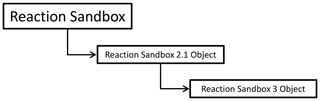Figure 2Schematic of a linked list of Reaction Sandboxes composed of two reaction objects instantiated from the class hierarchy shown in Fig. 1.

## 3.2 Implementation

The Reaction Sandbox is founded upon two files within the PFLOTRAN source code: reaction_sandbox_base.F90 and reaction_sandbox.F90.

### 3.2.1reaction_sandbox_base.F90

reaction_sandbox_base.F90 defines the base (or parent) Fortran class reaction_sandbox_base_type that the developer extends to create new (child) Reaction Sandbox classes. Code Block 1 illustrates the member variables and procedures within the reaction_sandbox_base_type class.This class contains a single member variable next, a pointer to the next class of the same reaction_sandbox_base_type type that enables the creation of an abstract linked list of Reaction Sandbox objects. The class also contains empty member procedures with prescribed subroutine interfaces (or argument lists) that the developer overrides in child classes. With the exception of BaseEvaluate, these procedures are empty and return immediately if not overridden by the child class. BaseEvaluate must be extended, and error messaging is incorporated within BaseEvaluate to ensure correct implementation. The other member procedures are optional and do not require implementation in the child classes. Appendix A documents the BaseXXX member procedure interfaces.

### 3.2.2reaction_sandbox.F90

reaction_sandbox.F90 serves as the main driver interface for the Reaction Sandbox, providing subroutines that manage the creation, reading, setup, execution and destruction of all Reaction Sandbox objects. For example, the subroutine RSandboxRead instantiates Reaction Sandbox objects based on the keywords parsed from the REACTION_SANDBOX block in the input file. RSandboxEvaluate calculates reaction rates by traversing the linked list of Reaction Sandboxes evaluating individual rates as shown in Code block 2 (subroutine argument lists have been omitted for simplicity).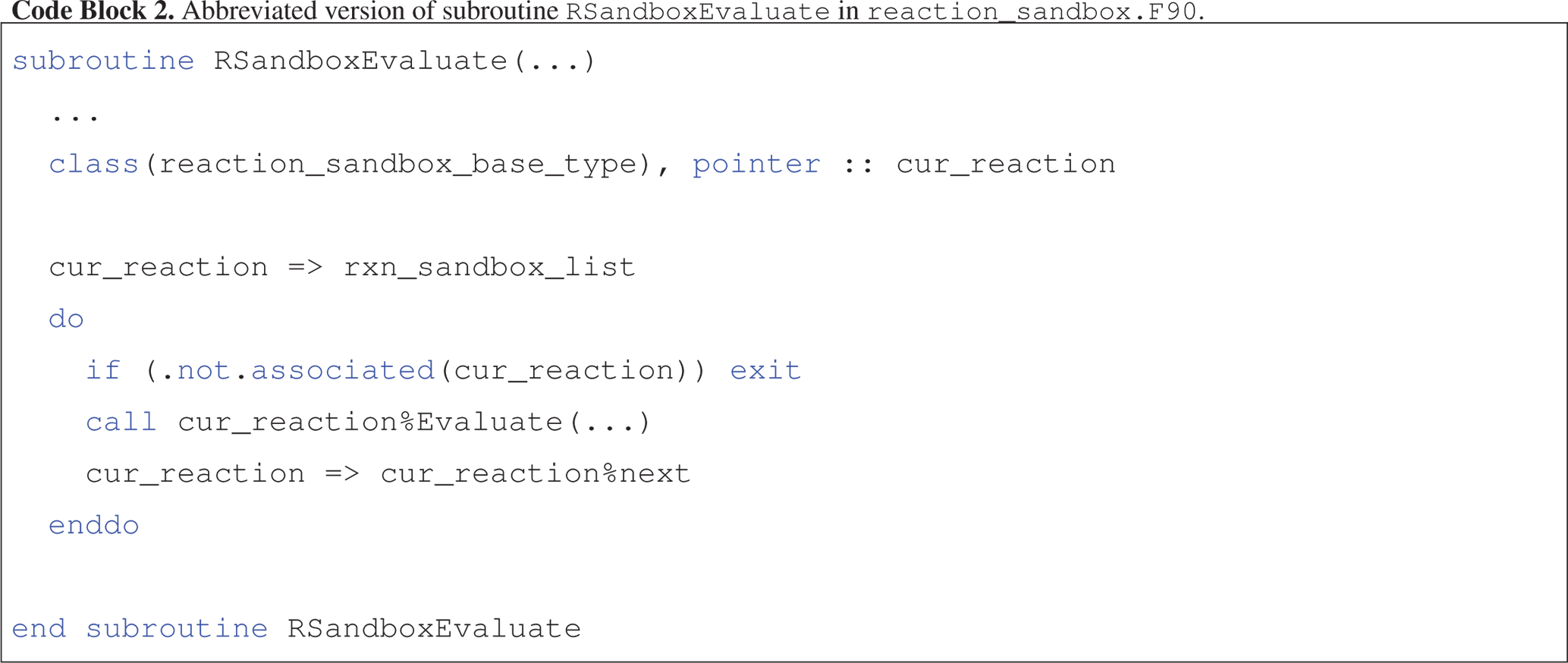With the exception of RSandboxRead, all member procedures are executed from within a linked list loop similar to that shown in Code block 2. Thus, subroutines within reaction_sandbox.F90 serve as interfaces to the linked lists of Reaction Sandbox objects, and all information is exchanged through these routines. The outer RSandboxXXX subroutines defined in reaction_sandbox.F90 may be called from other PFLOTRAN modules (e.g., see the call to RSandboxEvaluate within subroutine RReaction within reaction.F90), but member procedures within the Reaction Sandbox classes may not be called (to preserve data encapsulation).

The following section describes several example Reaction Sandboxes. These examples are implemented within the PFLOTRAN source code and may serve as templates for future sandboxes.

4 Example reaction sandboxes

This section illustrates the implementation of a few Reaction Sandboxes within PFLOTRAN. Reaction Sandbox Biodegradation Hill and Reaction Sandbox Flexible Biodegradation Hill were developed to demonstrate the implementation of a microbially mediated biodegradation reaction. Reaction Sandbox Simple provides a set of preconfigured reactions that may be uncommented, compiled and run to better understand kinetic rate expressions. Reaction Sandbox Example implements a first-order decay reaction. The comments within reaction_sandbox_example.F90 detail the steps necessary to implement a new Reaction Sandbox class.

### 4.1.1 Biodegradation conceptual model

Consider a microbially mediated biodegradation reaction with biomass growth and decay over time. The reaction could be expressed as

$\begin{array}{}\text{(R1)}& {A}_{\mathrm{aq}}+\mathrm{0.25}{B}_{\mathrm{aq}}\to \mathrm{0.33}{C}_{\mathrm{aq}}+{D}_{\mathrm{aq}},\end{array}$

with electron donor Aaq and acceptor Baq and products Caq and Daq [M]. The reaction is mediated by the immobile biomass species Xim [molebiomass m${}_{\text{bulk}}^{-\mathrm{3}}$] and inhibited above a Caq concentration of 10−4. The reaction rate Ir [mole m${}_{\text{bulk}}^{-\mathrm{3}}$ s−1 ] can be calculated using Michaelis–Menten kinetics as

$\begin{array}{}\text{(13)}& {I}_{\mathrm{r}}\phantom{\rule{0.33em}{0ex}}=\phantom{\rule{0.33em}{0ex}}{k}_{\text{max}}{X}_{\mathrm{im}}\frac{{A}_{\mathrm{aq}}}{{K}_{{A}_{\mathrm{aq}}}+{A}_{\mathrm{aq}}}×\frac{{B}_{\mathrm{aq}}}{{K}_{{B}_{\mathrm{aq}}}+{B}_{\mathrm{aq}}}×\frac{{I}_{{C}_{\mathrm{aq}}}}{{I}_{{C}_{\mathrm{aq}}}+{C}_{\mathrm{aq}}}\end{array}$

with maximum specific utilization rate constant kmax [mole mole${}_{\text{biomass}}^{-\mathrm{1}}$ s−1], half-saturation constants ${K}_{{A}_{\mathrm{aq}}}$ and ${K}_{{B}_{\mathrm{aq}}}$ [M], and inhibitor concentration ${I}_{{C}_{\mathrm{aq}}}$ [M]. The rate of biomass growth and decay can be modeled as

$\begin{array}{}\text{(14)}& \frac{\mathrm{d}X}{\mathrm{d}t}\phantom{\rule{0.33em}{0ex}}=\phantom{\rule{0.33em}{0ex}}yiel{d}_{{X}_{\mathrm{im}}}{I}_{\mathrm{r}}-{k}_{\text{decay}}X\end{array}$

with yield $yiel{d}_{{X}_{\mathrm{im}}}$ [molebiomass mole−1] and decay rate constant kdecay [s−1]. These rate expressions are implemented as microbial and biomass decay reactions within PFLOTRAN and enabled through the MICROBIAL_REACTION and IMMOBILE_DECAY_REACTION keywords in the CHEMISTRY block of the input file.

Figure 3 shows PFLOTRAN simulation results for an example batch experiment run over 7 d employing the reactions in Eqs. (13) and (14), reaction parameters in Table 2, stoichiometries in Reaction R1, and initial conditions in Table 3. Results plotted in the figure may be replicated through the following commands.

  cd $PFLOTRAN_DIR/regression_tests/ default/reaction_sandbox$PFLOTRAN_DIR/src/pflotran/pflotran
biodegradation-obs-0.pft

The plot shows the time evolution of aqueous and immobile species concentrations as the week-long simulation runs with a maximum time step size of 1 h. The results demonstrate that electron donor Aaq is the limiting substrate, as the concentrations for acceptor Baq and the reaction products Caq and Daq plateau when Aaq is nearly depleted at approximately 4 d. Biomass concentration increases through 2.25 d at which time the first-order decay rate exceeds the growth rate, and the population begins to fade. Superimposed on the figure are hypothetical experimental results for species Aaq (shown as circles) that deviate from the (blue) simulated curve in the log-scale plot. In this example, Eq. (13) is somewhat inaccurate in predicting the tailing behavior of species Aaq at late times.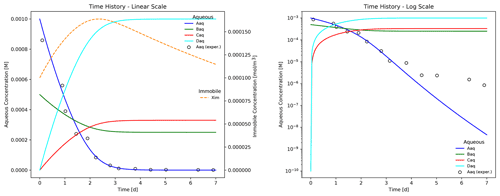Figure 3Time evolution of aqueous and immobile biomass species in a week-long batch biodegradation experiment.

Table 2Microbially mediated reaction parameters for the batch biodegradation experiment. n only applies to the reaction incorporating the Hill function (i.e., Eq. 15). M signifies molarity or mole per liter of water.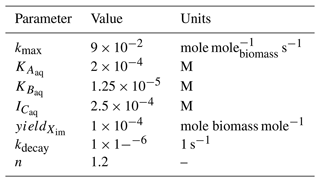The discrepancy in Aaq concentration can be resolved by employing a Hill function (Hill1910) within the Monod expression for Aaq. Here, the Aaq concentration and corresponding half-saturation constant are raised to the power n.

$\begin{array}{}\text{(15)}& {I}_{\mathrm{r}}\phantom{\rule{0.33em}{0ex}}=\phantom{\rule{0.33em}{0ex}}{k}_{\text{max}}{X}_{\mathrm{im}}\frac{{A}_{\mathrm{aq}}^{n}}{{K}_{{A}_{\mathrm{aq}}}^{n}+{A}_{\mathrm{aq}}^{n}}×\frac{{B}_{\mathrm{aq}}}{{K}_{{B}_{\mathrm{aq}}}+{B}_{\mathrm{aq}}}×\frac{{I}_{{C}_{\mathrm{aq}}}}{{I}_{{C}_{\mathrm{aq}}}+{C}_{\mathrm{aq}}}\end{array}$

However, the Hill function is not an option in PFLOTRAN; the code must be altered to accommodate this new feature. To further complicate matters, the researcher cannot modify the existing Monod expression within PFLOTRAN, since in doing so, the Hill function would be applied to both the Aaq and Baq Monod expressions. One possible solution is to implement Eq. (15) as a new reaction, and the Reaction Sandbox is designed to facilitate this process.

### 4.1.2 Reaction Sandbox Biodegradation Hill

Reaction Sandbox Biodegradation Hill implements the enhanced biodegradation reaction that incorporates the Hill function in Eq. (15) and the biomass growth and decay reaction in Eq. (14). The reactions are encoded in reaction_sandbox_biohill.F90. The reaction_sandbox_biohill_type class is presented in Code block 3, where integer IDs for each species are stored as class variables and the Setup and Evaluate procedures are redirected to local BioHillXXX implementations.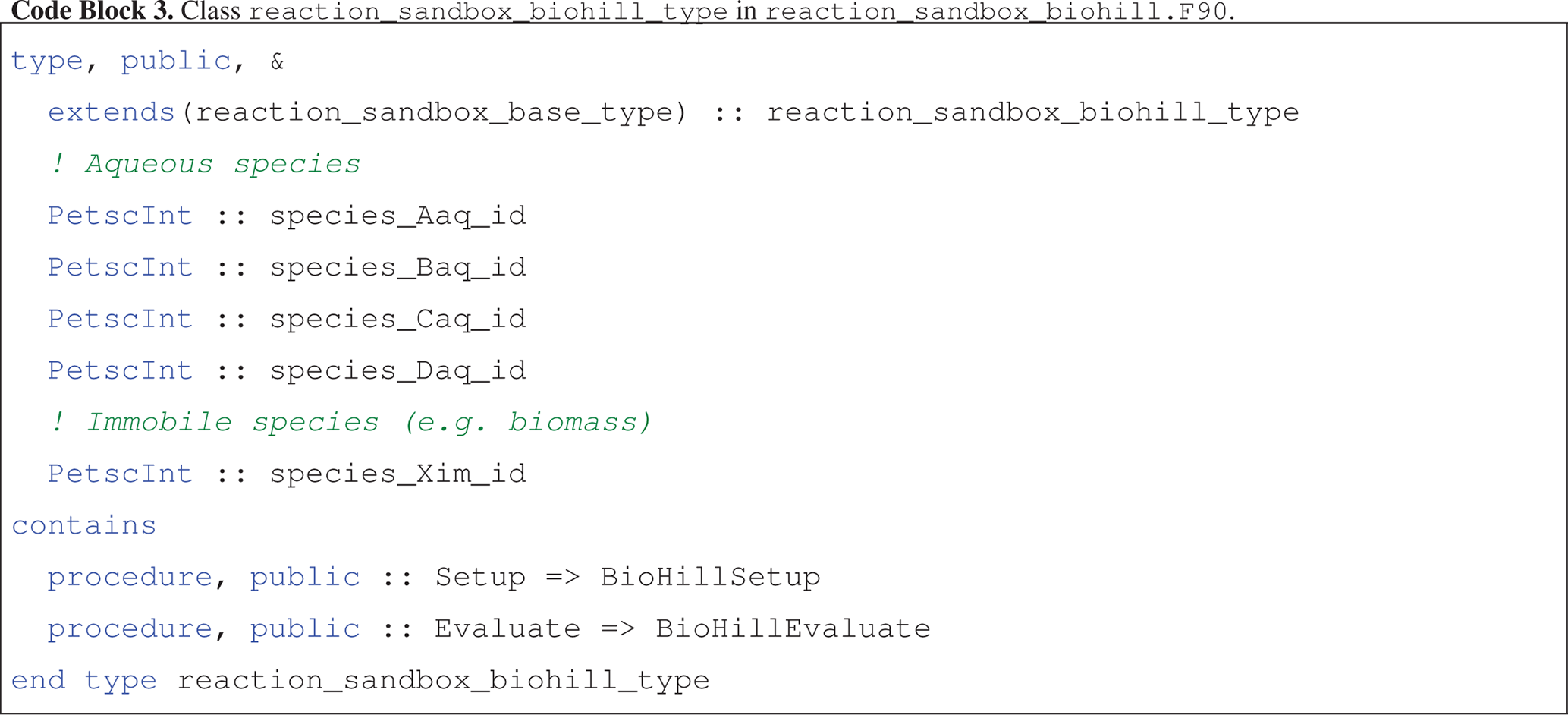Code block 4 illustrates the assignment of species IDs in an abbreviated version of BioHillSetup, based on keywords for aqueous species Aaq, Baq, Caq and Daq and immobile species Xim specified in the input file. BioHillEvaluate utilizes these IDs to access species concentrations and entries in the residual vector.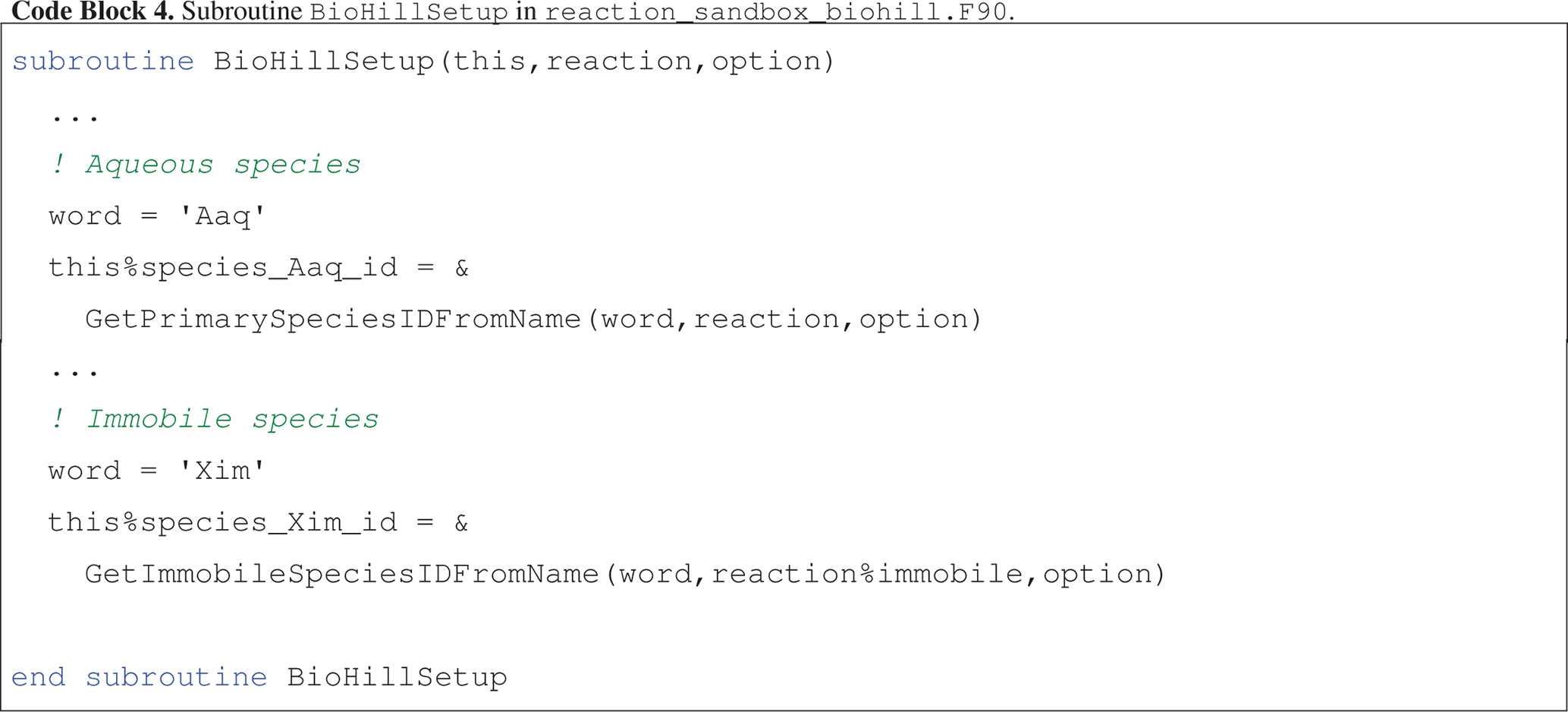Code block 5 presents an abbreviated version of the implementation of BioHillEvaluate where the naming convention for local variables corresponds closely to reaction parameters and state variables defined in Eqs. (14) and (15). Note that in the calculation of rate I_r, the concentration of Aaq and half-saturation constant K_Aaq are raised to the power n.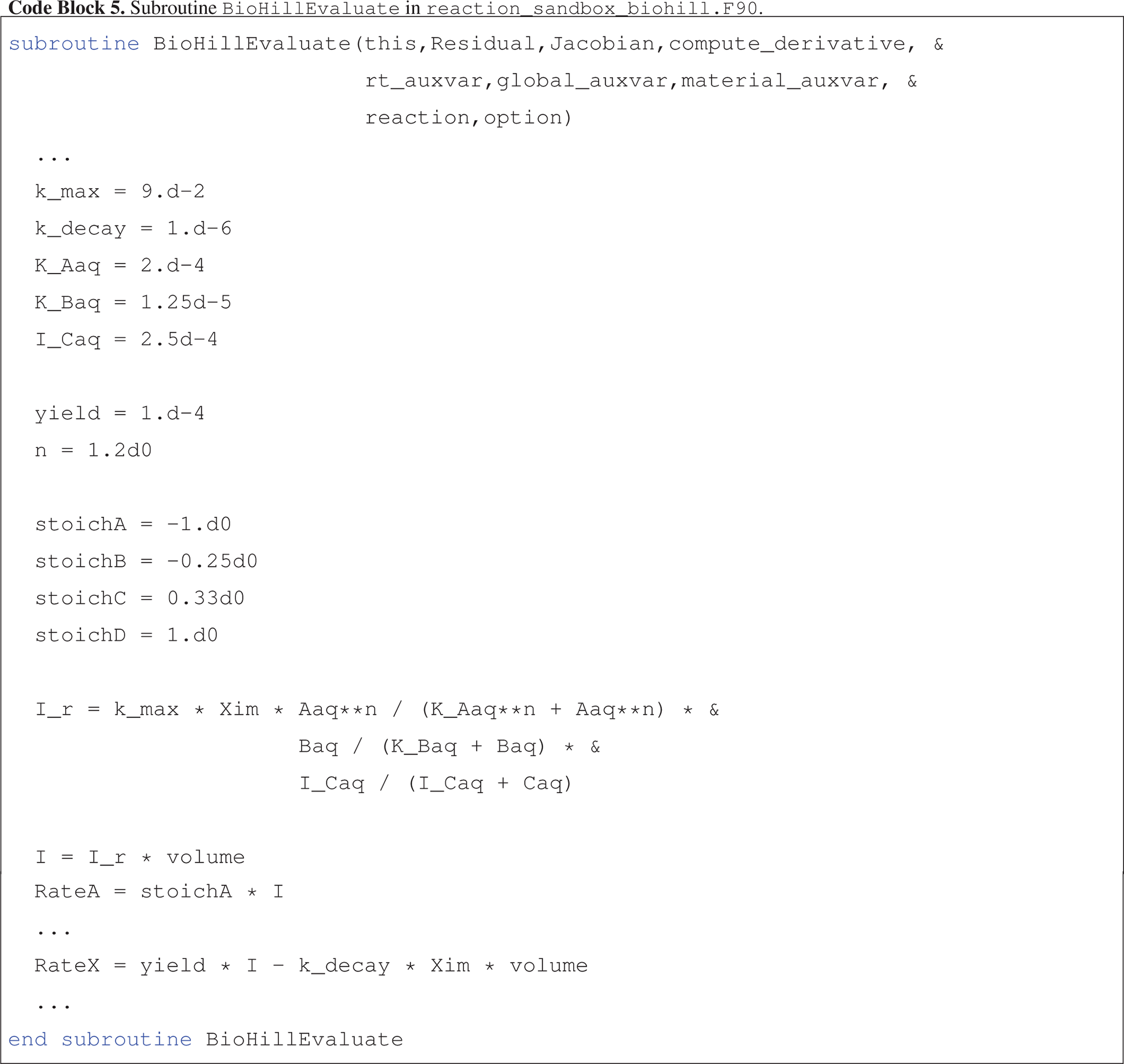Figure 4 illustrates a more accurate simulation result for the batch reaction experiment where the Hill function exponent n is set to 1.2. It is clear that the addition of the Hill function improves the model's ability to capture the tailing of species Aaq at low concentrations.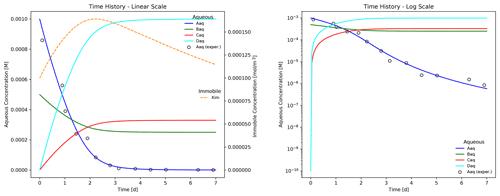Figure 4Improved match to the batch experiment results for species Aaq after incorporating the Hill function in Eq. (15). Compare with the log-scale plot (without the Hill function) in Fig. 3.

Results plotted in Fig. 4 may be replicated through the following commands:

  cd $PFLOTRAN_DIR/regression_tests/ default/reaction_sandbox$PFLOTRAN_DIR/src/pflotran/pflotran
biodegradation_hill-obs-0.pft

The implementation of the enhanced biodegradation reaction in Reaction Sandbox Biodegradation Hill is somewhat rigid. All reaction parameters (rate constants, half-saturation constants, stoichiometries, etc.) are hardcoded within the source code as shown in Code block 5 and may not be changed without code modifications. However, the implementation may be generalized, and this is demonstrated in Reaction Sandbox Flexible Biodegradation Hill.

### 4.1.3 Reaction Sandbox Flexible Biodegradation Hill

Reaction Sandbox Flexible Biodegradation Hill employs the same biodegradation, growth and decay reactions as Reaction Sandbox Biodegradation Hill with the added flexibility of specifying reaction parameters at run time through the input file, eliminating the need to re-compile PFLOTRAN every time a parameter changes. The new class is implemented in reaction_sandbox_flexbiohill.F90. The reaction_sandbox_flexbiohill_type class extends reaction_sandbox_biohill_type as presented in Code block 6. The child class inherits the species integer IDs from the parent and adds member variables for storing all reaction parameters and a logical flag for specifying the units of half-saturation constants K_Aaq and K_Baq and inhibitor concentration I_Caq (molality versus molarity). The dynamic stoich array enables the use of “do loops” in the Evaluate routine. The class redirects the ReadInput, Setup, Evaluate and Destroy procedures to local implementations, though only the implementation of FlexBioHillEvaluate will be described below.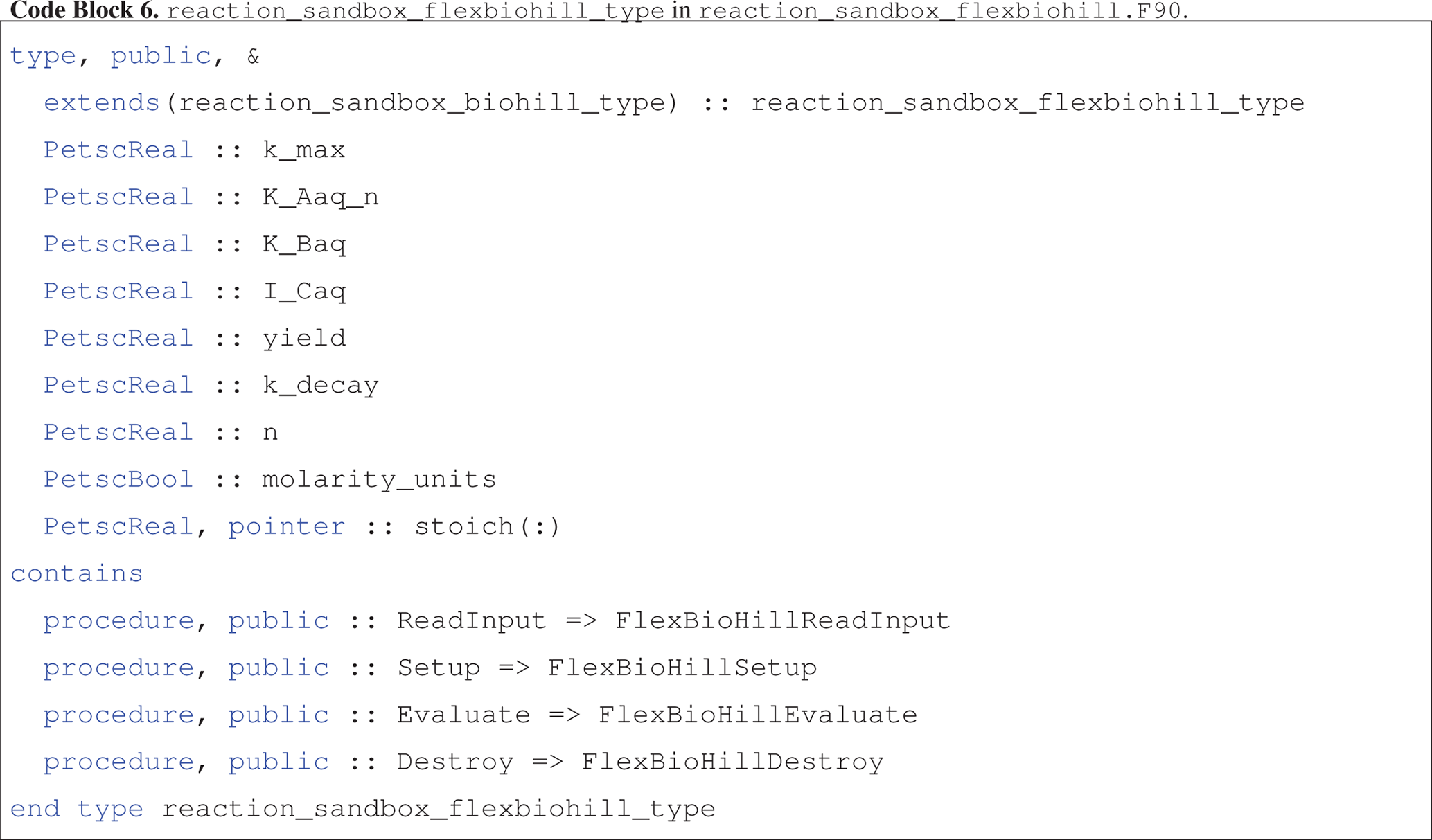The FlexBioHillEvaluate routine shown in Code block 7 is more succinct than BioHillEvaluate (Code block 5). Reaction parameters are no longer hardwired but read from the input file in FlexBioHillReadInput and stored as class member variables. The use of the class member stoich array within the do loops eliminates the need to hardwire the Residual array indexing and reduces the number of lines of code. In addition, FlexBioHillEvaluate demonstrates the ability to choose between molality versus molarity for aqueous concentrations and half-saturation constants, a useful option since laboratory data are often available in either format. There is also support for calculating analytical derivatives (for the Jacobian), which can be programmed much more concisely with the stoich array and do loops (not shown).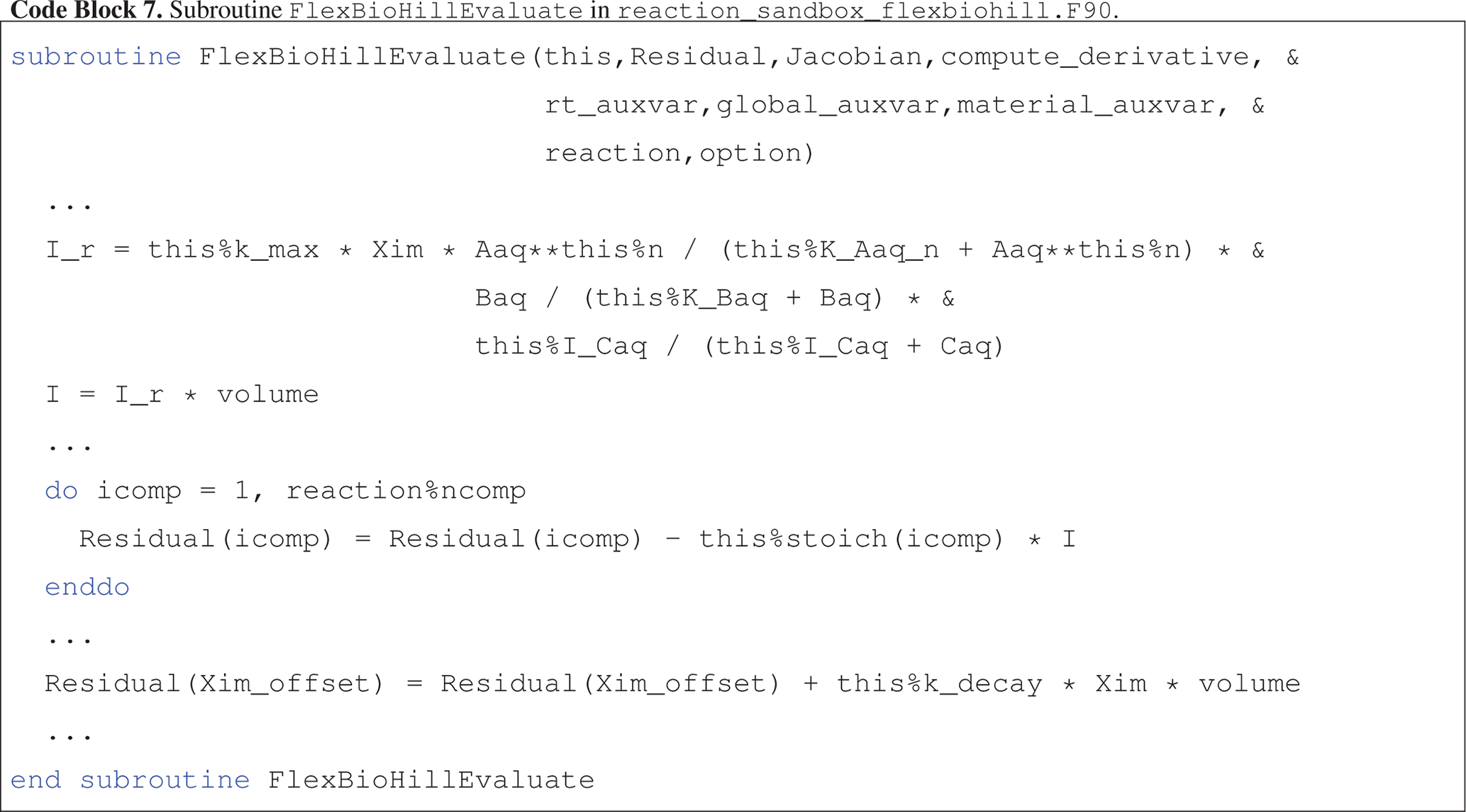Flexible Biodegradation Hill produces results identical to Biodegradation Hill when given identical initial conditions and Biodegradation Hill's reaction parameters. These results may be compared by running the script compare_biodegradation_results.py after completing both simulations, as follows.

  cd $PFLOTRAN_DIR/regression_tests/ default/reaction_sandbox$PFLOTRAN_DIR/src/pflotran/pflotran
$PFLOTRAN_DIR/src/pflotran/pflotran -input_prefix flexible_biodegradation_hill python compare_biodegradation _results.py ## 4.2 Reaction Sandbox Simple Reaction Sandbox Simple provides a framework for evaluating reactive transport in 1D using common kinetic rate expressions with a preconfigured set of six aqueous and two immobile species (Aaq, Baq, Caq, Daq, Eaq, Faq, Xim, Yim). The conceptual model consists of a 100 m, liquid-saturated column with a Dirichlet boundary condition at the inlet (x=0 m) and a zero-gradient boundary condition at the outlet (x=100 m). Grid spacing is set to 1 m resolution. The prescribed Darcy velocity is 1 m yr−1 with a pore water velocity of 4 m yr−1 (porosity = 0.25). Throughout the simulation, solutes enter at the inlet and react within the domain. Simulation results are stored in two formats: (1) snapshots of the entire domain at select times and (2) continuous observation at a mid-column observation point (x=49.5 m). Table 4 summarizes simulation parameters, while Table 5 describes the initial and boundary concentrations. Table 5Initial and boundary concentrations in the Reaction Sandbox Simple input file.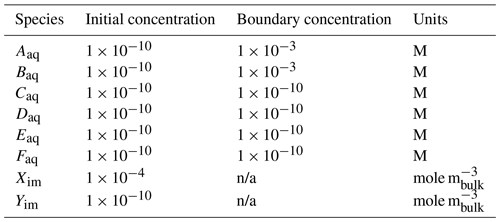n/a – not applicable The implementation of the reaction_sandbox_simple_type class is nearly identical to that of reaction_sandbox_biohill_type in Code block 3 with the addition of member variables species_Eaq_id, species_Faq_id and species_Yim_id. Member procedure SimpleSetup links these integer IDs to their respective names from the input file. The user may evaluate reactions in this sandbox with the following steps: 1. Uncomment a rate expression block within subroutine SimpleEvaluate. • cd$PFLOTRAN_DIR/src/pflotran

•

[emacs,gedit,nano,vi] reaction_sandbox_simple.F90

•

Remove “!uncomment:” prefixes from lines within chosen rate expression in subroutine SimpleEvaluate.

•

Save the file.

2. Compile the PFLOTRAN executable.

•

make pflotran

3. Navigate to $PFLOTRAN_DIR/regression_tests/ default/reaction_sandbox. • cd$PFLOTRAN_DIR/regression_tests/ default/reaction_sandbox

4. Change the x-direction grid resolution in reaction_sandbox_simple.in to 100.

•

[emacs,gedit,nano,vi] reaction_sandbox_simple.in

•

NXYZ 10 1 1 NXYZ 100 1 1

•

Save the file.

5. Run the simulation.

•

$PFLOTRAN_DIR/src/pflotran/pflotran -input_prefix reaction _sandbox_simple 6. Plot the results with reaction_sandbox_simple.py. • python reaction_sandbox_simple.py Code block 8 illustrates a commented rate expression block for calculating the first-order decay of species Aaq to daughter product Caq.The user deletes all “!uncomment:” prefixes in the code block as shown in Code block 9, compiles PFLOTRAN, navigates to the reaction_sandbox folder and runs the code.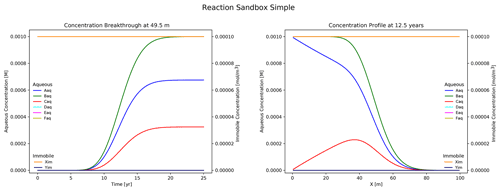Figure 5Results from Reaction Sandbox Simple with the first-order A C rate expression uncommented. Download Figure 5 illustrates the simulation results. Plotted to the left is concentration breakthrough at the observation point and to the right is a snapshot of concentration profiles at 12.5 years. Species Aaq clearly decays into Caq while the other species are transported without reaction. The user may evaluate the other rate expressions in the subroutine with the same approach. ## 4.3 Reaction Sandbox Example PFLOTRAN's reaction_sandbox_example.F90 serves as a template for implementing new Reaction Sandboxes. The sandbox implements the first-order decay of Aaq without daughter products, and the developer modifies the source code template to incorporate new reactions. Comments within the source code enumerate steps for implementing new reactions beginning with the renaming of subroutines and variables and ending with the implementation of subroutine ExampleDestroy at the bottom of the file. In between, source code is modified to implement the new reaction(s). Code block 10 illustrates the first two steps embedded within comments in the source code near the top of the file.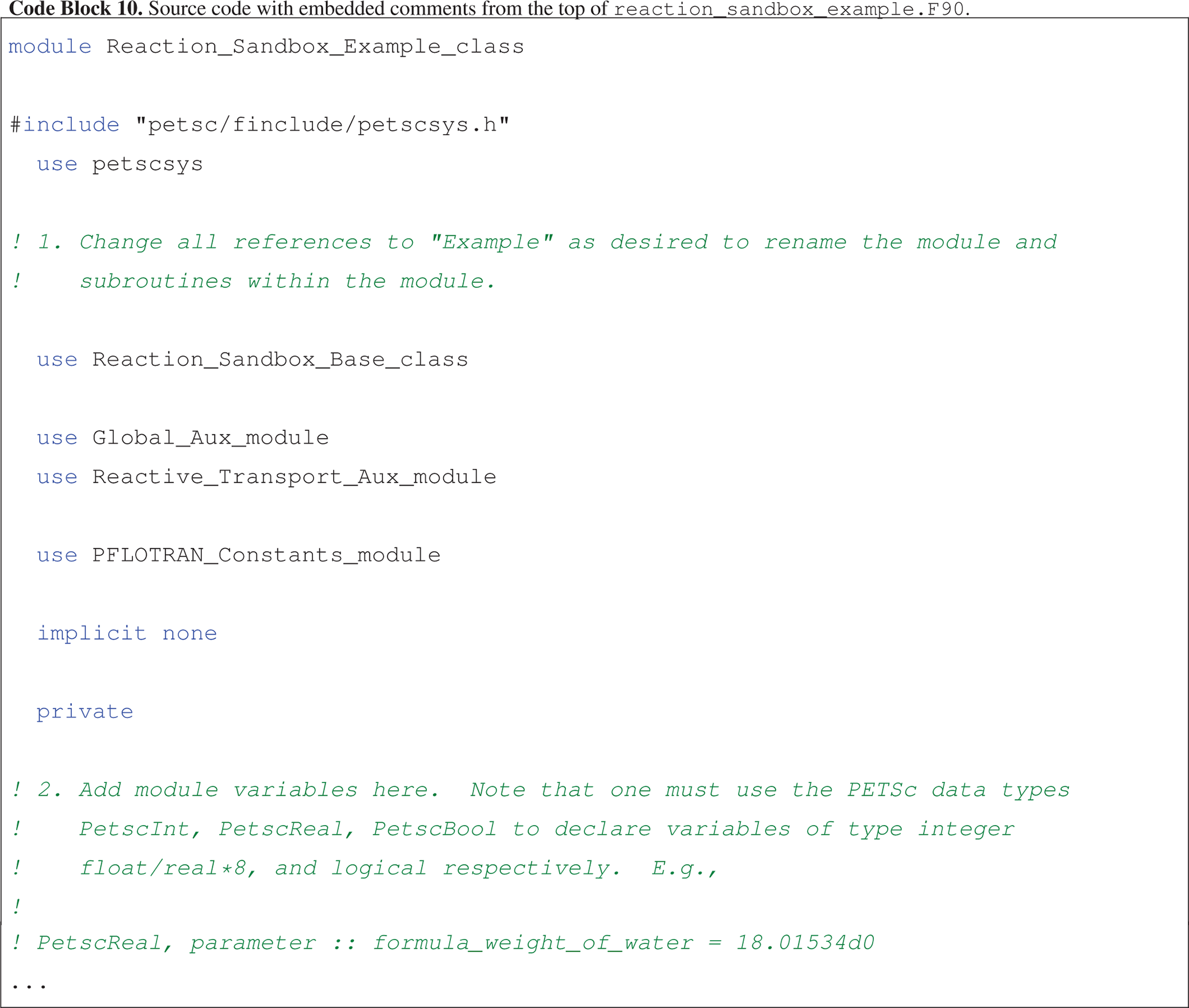Comments within ExampleEvaluate provide a detailed description of the subroutine's arguments and local variables, including members of the reaction and reactive transport auxiliary variable classes (i.e., reaction_rt_type and reactive_transport_auxvar_type, respectively) which may be used in rate expression calculations. The units of all variables and the Residual and Jacobian arrays are also provided. Code block 11 shows several of these detailed comment blocks.Once refactoring is complete, the developer must modify the corresponding class and subroutine names within reaction_sandbox.F90 to match those in the newly refactored reaction_sandbox_example.F90. The source code may then be compiled without changing the reaction_sandbox_example.F90 filename. Should this filename be revised, the developer must update the corresponding filenames within the pflotran_object_files.txt and pflotran_dependencies.txt files referenced by the makefile. The best approach to compiling the code is to perform a make clean to remove all previously built modules and object files and the pflotran executable and then make pflotran to compile the code. reaction_sandbox_example.in, located in$PFLOTRAN_DIR/regression_tests/default/ reaction_sandbox, provides a representative input deck for this example. Note that the card EXAMPLE in the REACTION_SANDBOX block of this input file must be updated to match the corresponding keyword added to reaction_sandbox.F90.

5 Conclusions

Customization of biogeochemical reaction networks is often necessary in the development of reactive transport simulators employed to simulate problem-specific scenarios in the real world. For researchers in the natural sciences, who may be more focused on biology, chemistry and/or physics than computational science, modifying these codes to incorporate new scientific processes can be challenging. The PFLOTRAN Reaction Sandbox may help remedy this issue.

The Reaction Sandbox provides a modular environment for prototyping new kinetic rate expressions that do not exist within PFLOTRAN. Within the Reaction Sandbox, novel reaction networks can evolve and mature over time; natural selection can run its course. Once vetted, these reactions may be incorporated more efficiently elsewhere within the permanent code base.

This work demonstrates the implementation of reactions within the Reaction Sandbox. Several new Reaction Sandbox classes are conceptualized and implemented based on existing rate expressions within PFLOTRAN for microbially mediated biodegradation to improve the code's ability to match hypothetical empirical data and provide greater flexibility from the end-user perspective. Reaction Sandbox Simple is presented as a means of prototyping numerous common kinetic rate expressions within a preconfigured column experiment context. Reaction Sandbox Example provides a template for implementing new reactions within PFLOTRAN.

Appendix A: Reaction sandbox member procedure interfaces

This section documents Fortran class reaction_sandbox_base_type member procedure interfaces not covered in Section 3.2.1 (see reaction_sandbox_base.F90).

## A1ReadInput => BaseReadInput

ReadInput (Code block 12) provides a customizable interface for reading parameters associated with the reaction sandbox from a block in the input file. The input block is opened by a unique keyword associated with the child Reaction Sandbox class.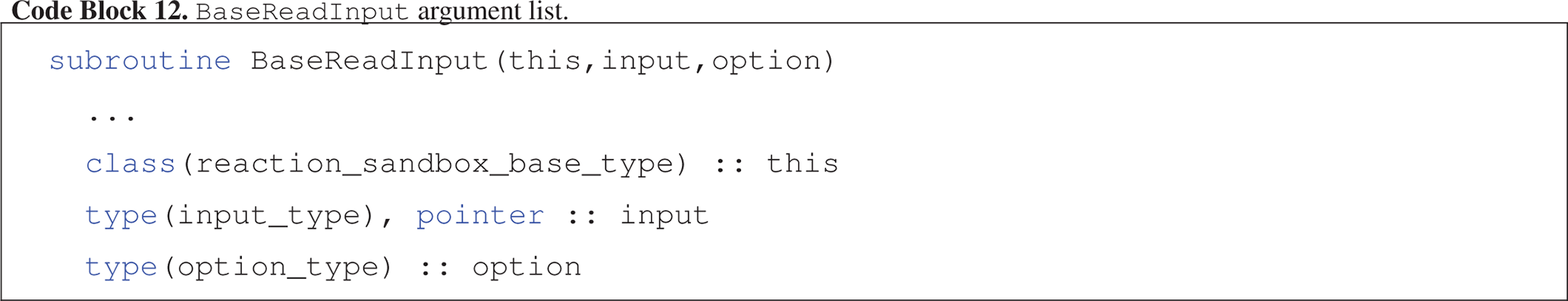•

this : reaction_sandbox_base_type object

•

input : object storing the input file object pointer and line buffers

•

option : object storing run time options including process rank and an error messaging buffer

## A2Setup => BaseSetup

Setup (Code block 13) initializes the Reaction Sandbox class by allocating dynamic memory, mapping species IDs, assigning stoichiometries and rate constants, etc. The degree to which Reaction Sandbox class settings are customizable at run time is up to the developer and their creativity in implementing ReadInput and Setup.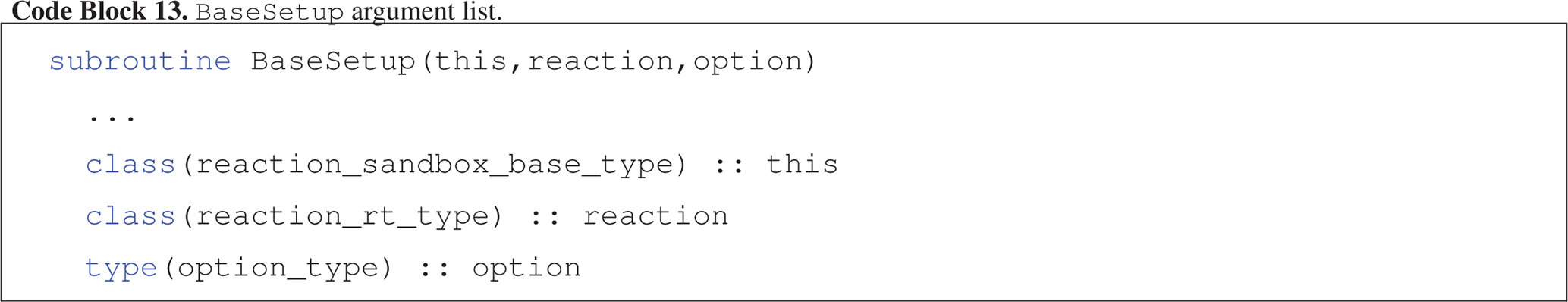•

this : reaction_sandbox_base_type object

•

reaction : object storing chemical species and general reaction information

•

option : object storing run time options including process rank and an error messaging buffer

## A3Evaluate => BaseEvaluate

Evaluate (Code block 14) calculates kinetic rates for all reactions in the Reaction Sandbox class and adds the (kinetic rate) contributions to the Residual and Jacobian arrays. This is the only subroutine that must be extended in child Reaction Sandbox classes.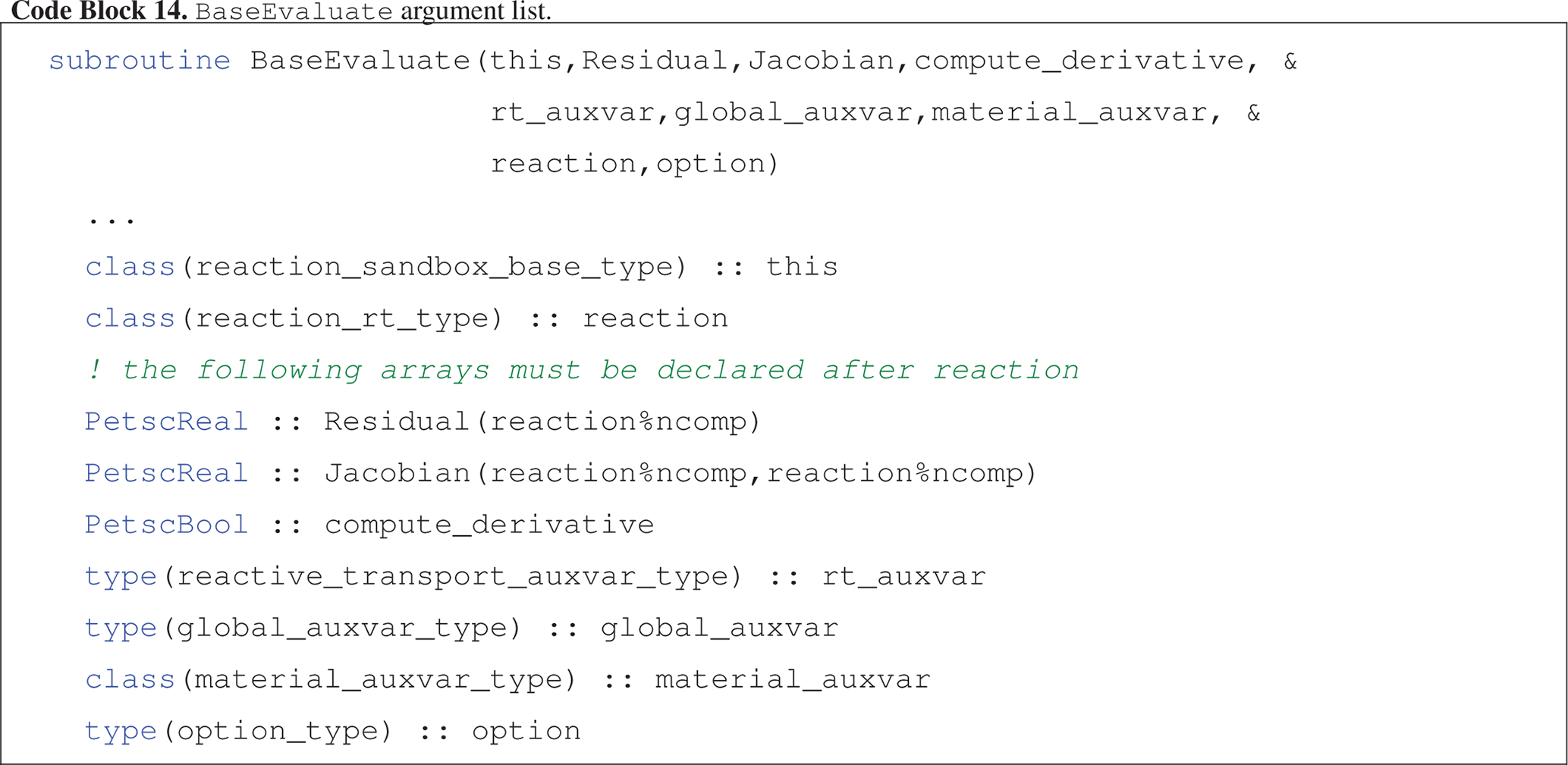•

this : reaction_sandbox_base_type object

•

Residual : 1D array of double precision numbers holding contributions to the residual equations at each grid cell

•

Jacobian : 2D array of double precision numbers holding contributions to the Jacobian matrix at each grid cell

•

compute_derivative : flag toggling on the calculation of analytical derivatives for the Jacobian matrix when true

•

rt_auxvar : object storing reactive transport state variables (e.g., concentrations, rates) at each grid cell

•

global_auxvar : object storing flow state variables (e.g., density, saturation) at each grid cell

•

material_auxvar : object storing material and cell properties (e.g., porosity, volume) at each grid cell

•

reaction : object storing chemical species and general reaction information

•

option : object storing run time options including process rank and an error messaging buffer

## A4UpdateKineticState => BaseUpdateKineticState

UpdateKineticState (Code block 15) updates state variables associated with the Reaction Sandbox class that are stored in rt_auxvar and updated at the end of a time step based on rates calculated in the Reaction Sandbox (e.g., for mass balance calculations, updates to mineral volume fractions).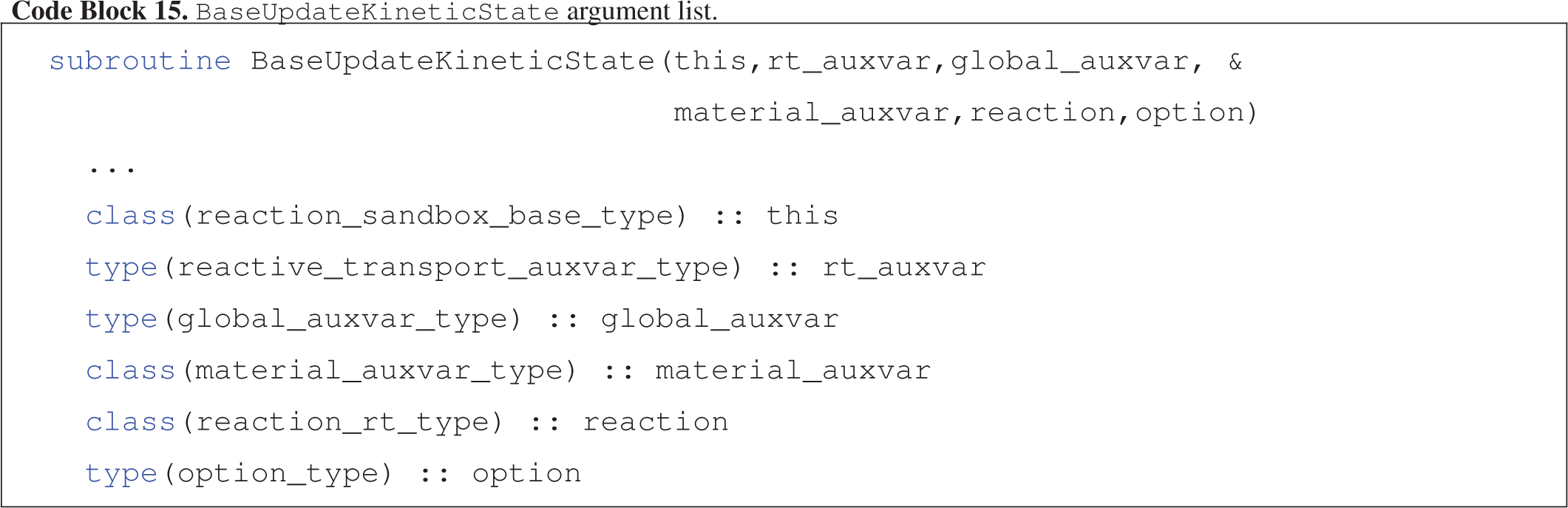•

this : reaction_sandbox_base_type object

•

rt_auxvar : object storing reactive transport state variables (e.g., concentrations, rates) at each grid cell

•

global_auxvar : object storing flow state variables (e.g., density, saturation) at each grid cell

•

material_auxvar : object storing material and cell properties (e.g., porosity, volume) at each grid cell

•

reaction : object storing chemical species and general reaction information

•

option : object storing run time options including process rank and an error messaging buffer

## A5AuxiliaryPlotVariables => BaseAuxiliaryPlotVariables

AuxiliaryPlotVariables (Code block 16) appends Reaction Sandbox-specific state variables stored in rt_auxvar to the list of output variables to be printed to observation and snapshot files.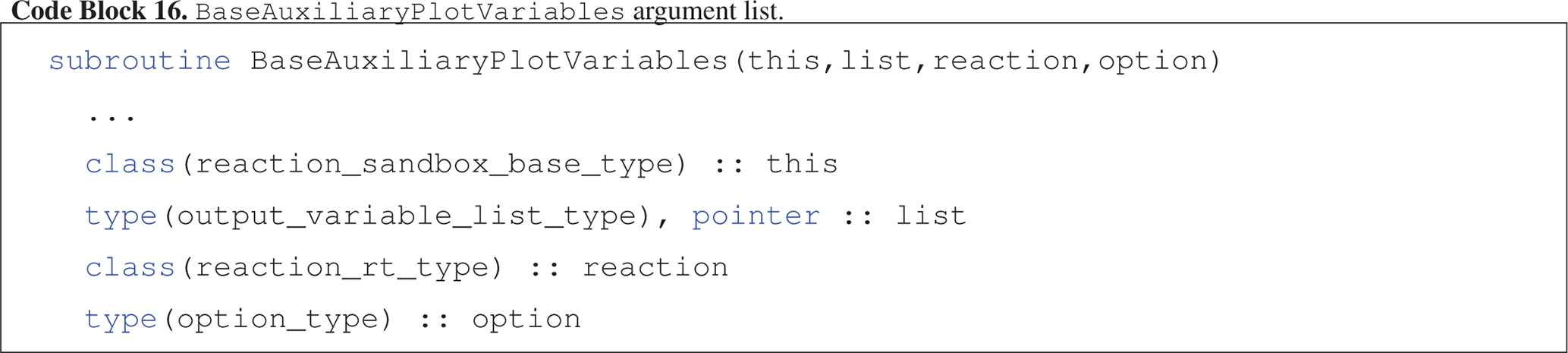•

this : reaction_sandbox_base_type object

•

list : output_variable_list_type object storing a linked list of sandbox-specific output_variable_type objects

•

reaction : object storing chemical species and general reaction information

•

option : object storing run time options including process rank and an error messaging buffer

## A6Destroy => BaseDestroy

Destroy (Code block 17) deallocates all dynamic memory in the Reaction Sandbox class at the end of a simulation.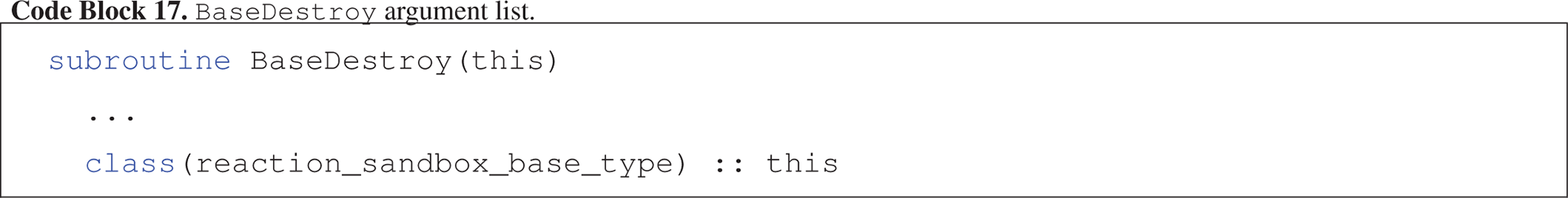•

this : reaction_sandbox_base_type object

Code availability

The source code, input files and results presented in this manuscript are based on PFLOTRAN v4.0. A snapshot of this release is available at https://doi.org/10.5281/zenodo.5826289 (Hammond2022). The corresponding version of PETSc is v3.16.2 and was configured on Ubuntu 18.04 with GCC 7.5 using the following config script: ./configure --CFLAGS='-O3' --CXXFLAGS='-O3' --FFLAGS='-O3' --with-debugging=no --download-mpich=yes --download-hdf5=yes --download-hdf5-fortran-bindings=yes --download-fblaslapack=yes --download-metis=yes --download-parmetis=yes.

Competing interests

The contact author has declared that there are no competing interests.

Disclaimer

Publisher's note: Copernicus Publications remains neutral with regard to jurisdictional claims in published maps and institutional affiliations.

Acknowledgements

This research was supported by the U.S. Department of Energy (DOE), Office of Biological and Environmental Research (BER), as part of BER's Environmental System Science Program (ESS). This contribution originates from the River Corridor Scientific Focus Area (SFA) at the Pacific Northwest National Laboratory (PNNL) and was supported by the partnership with IDEAS-Watersheds. PNNL is operated for the DOE by Battelle Memorial Institute under contract DE-AC05-76RL01830. This paper describes objective technical results and analysis. Any subjective views or opinions that might be expressed in the paper do not necessarily represent the views of the U.S. Department of Energy or the United States Government.

Financial support

This research has been supported by the Office of Science (grant no. DE-AC05-76RL01830). ​​​​​​​

Review statement

This paper was edited by Richard Mills and reviewed by Allan Leal and Bhavna Arora.

References

Andre, B., Bisht, G., Collier, N., Frederick, J., Hammond, G., Jaysaval, P., Karra, S., Kumar, J., Leone, R., Lichtner, P., Mills, R., Nole, M., Orsini, P., Park, H., and Rousseau, M.: PFLOTRAN Theory Guide, http://documentation.pflotran.org, last access: 23 February 2022. a

Bethke, C. M.: Geochemical and Biogeochemical Reaction Modeling, Cambridge University Press, 2nd Edn., https://doi.org/10.1017/CBO9780511619670, 2007. a

Chapman, S.: Fortran for Scientists & Engineers, McGraw-Hill Higher Education, 4th Edn., ISBN10: 0073385891, ISBN13: 9780073385891, 2018. a

Hammond, G.: PFLOTRAN Reaction Sandbox, Zenodo [code], https://doi.org/10.5281/zenodo.5826289, 2022. a

Hammond, G. E., Lichtner, P. C., and Mills, R. T.: Evaluating the performance of parallel subsurface simulators: An illustrative example with PFLOTRAN, Water Resour. Res., 50, 208–228, https://doi.org/10.1002/2012WR013483, 2014. a

Hill, A. V.: The possible effects of the aggregation of the molecules of hæmoglobin on its dissociation curves, J. Physiol., 40, i–vii, 1910. a

Lichtner, P. C.: Continuum model for simultaneous chemical reactions and mass transport in hydrothermal systems, Geochim. Cosmochim. Ac., 49, 779–800, https://doi.org/10.1016/0016-7037(85)90172-3, 1985. a

Liu, C., Zachara, J. M., Qafoku, N. P., and Wang, Z.: Scale-dependent desorption of uranium from contaminated subsurface sediments, Water Resour. Res., 44, W08413, https://doi.org/10.1029/2007WR006478, 2008. a

Rubin, J.: Transport of reacting solutes in porous media: Relation between mathematical nature of problem formulation and chemical nature of reactions, Water Resour. Res., 19, 1231–1252, https://doi.org/10.1029/WR019i005p01231, 1983. a

Steefel, C., Appelo, C., Arora, B., Jacques, D., Kalbacher, T., Kolditz, O., Lagneau, V., Lichtner, P. C., Mayer, K. U., Meeussen, J. C. L., Molins, S., Moulton, D., Shao, H., Simunek, J., Spycher, N., Yabusaki, S. B., and Yeh, G. T.: Reactive transport codes for subsurface environmental simulation, Comput. Geosci., 19, 445–478, https://doi.org/10.1007/s10596-014-9443-x, 2015. a

Steefel, C. I., DePaolo, D. J., and Lichtner, P. C.: Reactive transport modeling: An essential tool and a new research approach for the Earth sciences, Earth Planet. Sc. Lett., 240, 539–558, https://doi.org/10.1016/j.epsl.2005.09.017, 2005.  a

Tutolo, B. M., Luhmann, A. J., Tosca, N. J., and Seyfried Jr., W. E.: Serpentinization as a reactive transport process: The brucite silicification reaction, Earth Planet. Sc. Lett., 484, 385–395, https://doi.org/10.1016/j.epsl.2017.12.029, 2018. a